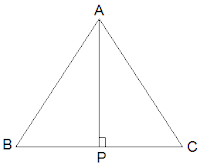Newbie

# ABC is an isosceles triangle with AB = AC. Draw AP ⊥ BC to show that B = C. Q.5

• 0

This is very important question of ncert class 9th of chapter triangles. How I solve the best solution of exercise 7.3 question number 5. Please help me to solve this in a easy and best way.ABC is an isosceles triangle with AB = AC. Draw AP ⊥ BC to show that B = C.

Share

1. Solution:In the question, it is given that AB = AC

Now, ΔABP and ΔACP are similar by RHS congruency as

APB = APC = 90° (AP is altitude)

AB = AC (Given in the question)

AP = AP (Common side)

So, ΔABP ΔACP.

∴ B = C (by CPCT)

• 0